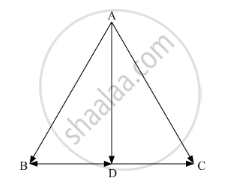# The two vectors j+k and 3i−j+4k represent the two sides AB and AC, respectively of a ∆ABC. Find the length of the median through A - Mathematics

The two vectors hatj+hatk " and " 3hati-hatj+4hatk represent the two sides AB and AC, respectively of a ∆ABC. Find the length of the median through A

#### Solution

In ABC,Using the triangle law of vector addition, we have

vec(BC)=vec(AC)-vec(AB)

=(3hati-hatj+4hatk)-(hatj+hatk)

=3hati-2hatj+3hatk

:.vec(BD)=1/2vec(BC)=3/2hati-hatj+3/2hatk " (Since AD is the median)"

In ∆ABD, using the triangle law of vector addition, we have

vec(AD)=vec(AB)+vec(BD)

=(hatj+hatk)+(3/2hati-hatj+3/2hatk)

=3/2hati+0hatj+5/2hatk

:.AD=sqrt((3/2)^2+0^2+(5/2)^2)=1/2sqrt34

Hence, the length of the median through A is 1/2sqrt34units.

Concept: Position Vector of a Point Dividing a Line Segment in a Given Ratio
Is there an error in this question or solution?